# Test Case

## Description of the study test case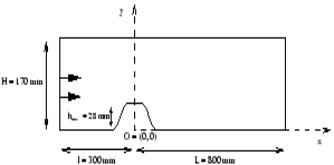Figure 1: 2D single hill flow.

The case considered is the first configuration described in Almeida et al. (1993), a single hill mounted on the bottom of the channel. In the second case consisting of the flow over a series of consecutive hills mounted at the same location is not considered as it was shown at the workshop that the number of hills (10) was not sufficient to assume periodic calculations, and furthermore 3D effects were suspected. The channel height is H = 170 mm and the maximum height and length of each hill are respectively hmax = 28 mm and 2R = 108 mm. In the case of consecutive hills, the space between each of them is 4.5hmax. The shape of the hills was to be the inverse of a fourth-order polynomial but the actual shape is somewhat different. The actual values of the height h(x) of the two-dimensional hills are stored on the ERCOFTAC/Classic Collection website (http://cfd.mace.manchester.ac.uk/ercoftac).

### Flow Parameters

• Water with a kinematic viscosity: ν = 1×10-6 m2/s.
• Mean centreline velocity at inlet: Uo = 2.147 m/s.
• Reynolds number: Uoh/ν = 60,000.

### Inflow Conditions

Measurements are provided for a fully-developed channel flow in the absence of the hill(s) and at the same location. The centreline turbulence intensity is about 3%, the friction factor is Cf = 0.0027 and the wall friction velocity is u* = 0.079 m/s. The profiles of mean velocity and Re stresses are available in the database, but are not very different from fully-developed channel flow for which a precursor simulation or analytical profiles can be used. These analytical profiles are given in section 5.2.2

## Test Case Experiments

Almeida et al. (1993) carried out velocity measurements using a Laser-Doppler Velocimeter up to 2 mm from the surface of the hill(s) and the bottom of the channel. For a 95% confidence level the error is estimated at 0.5% for mean velocity and 3% for Reynolds stresses.

Available Measurements

Mean velocity and Reynolds stresses are given along 14 cross-stream profiles and available both in the ERCOFTAC database (via http://www.ercoftac.org/ then "databases" and "classic collection" or directly via this link.

## CFD Methods

Before describing recent CFD results we recall that in 1995 this test case was analysed at an ERCOFTAC /IAHR workshop. The wide range of methods and models used resulted in an equally wide range of dispersion of the results. This is recalled here to illustrate that the test case is quite challenging. Samples of streamline plots are shown on Figure 2 and some of the conclusions reported by Rodi et al. (1998) are repeated herein (as this EDF report was not widely distributed). Detailed profiles using the so-called reference solutions will be presented in the next section.

5.1 Karslruhe 1995 workshop

Altogether 38 different results were submitted for the Karlsruhe Workshop. Six computor groups submitted results obtained with the standard k-ε model using standard wall functions. The separation was predicted slightly too late and reattachment considerably too early so that the separation zone is too short by 25 to 40%. The use of the RNG version of the k-ε model produces a larger separation region, which is in fact too large. Results obtained with two-layer models combining the standard k-ε model with various one-equation models near the wall yielded generally good agreement among the various calculations and come closer to the experimental picture. When the RNG k-ε model is combined with a one-equation near-wall model, the recirculation length is much too large. Four different low-Re k-ε models were used which give results similar to those obtained with the two-layer models, agreeing fairly well among each other and with the experiments. Only the Lam-Bremhorst version produces a too long separation zone. The conclusion that can be drawn here is that the prediction of the separation zone can vary significantly with the type of wall function implementation, and of course influences the size of the recirculation bubble.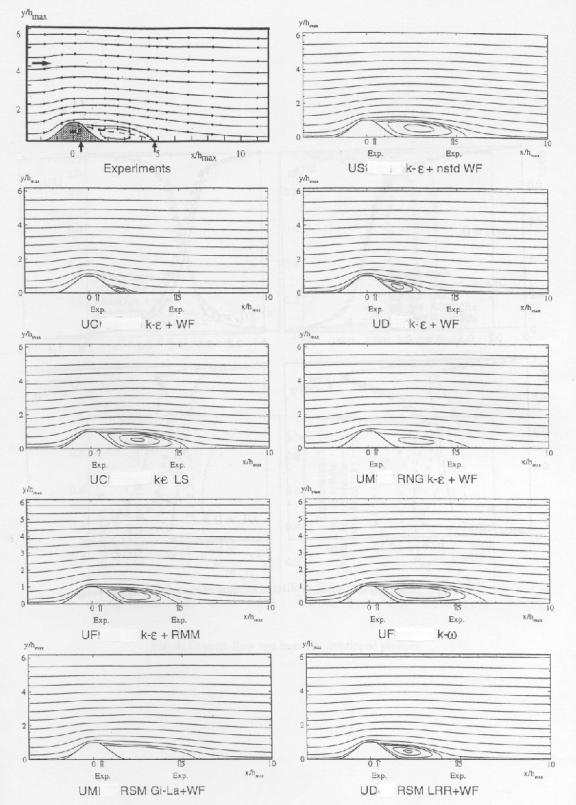Figure 2: Streamlines with typical models compared at the Karslruhe Workshop (From Rodi et al. 1998)

With RSM, the separation zone is predicted longer than with the k-ε model but still somewhat shorter than experimentally observed. When the Gibson-Launder (1978) Reynolds-stress model is used (i.e. adding « wall echo terms »), the recirculation length is over-predicted (either with wall functions or with a one-equation near-wall model).

In the lee side of the hill, all models under-predict the level of k and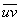in the separated shear layer. The Reynolds-stress models produced generally rather lower values of k andFurther downstream, after reattachment, the U-velocity distribution is predicted fairly well by most k-ε-based models, the peak being somewhat closer to the lower wall than in the experiments. Here the k-ω models and also some of the Reynolds-stress models develop larger differences. As the peaks in kandbecome lower further downstream, these levels are better picked up by most models. The standard k-ε model with wall functions still under-predicts the peaks at the downstream station. The k-ω model produces excessive k- and-levels further downstream, while most Reynolds-stress models still under-predict the levels.

5.2 Recent Simulations (2001)

Using the hill flow test case, several students were given the task of computing this case with different codes at their disposal; some at EDF R&D Paris, and some at UMIST Manchester. Until the final stage, each student was asked to establish his own "best converged" solution independently, using for this assessment text-book rules such as checking grid independence, convection schemes, inlet conditions. Only at the end did they actually compare their solutions and fortunately these now coincided. Of the 4 codes used in this exercise two are major commercial codes and two "in house" well validated codes. All use finite volumes, and in this case only structured grids (one of them staggered, 3 others collocated). The names of the codes and their specific features are irrelevant since the purpose here is to establish a reference solution" for each turbulence model, not to compare advantages and weaknesses of these codes.

This common solution produced represents the true solution to e.g. the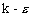model, independently from the numerical methods or even the specific grids (as long as they are fine enough). This reference solution is included in the ERCOFTAC database alongside the experimental data from which it inevitably differs. It can then be used in validation procedures of new codes. The exercise then consists in recovering this reference solution for a given model, and not to match the experimental data as close as possible. Only when this is achieved can discussions on "improvements" of the turbulence models be considered.

The 4 codes will be designed by the letters C, E, F and G. Grid refinement effects were tested independently with E and F.

5.2.1 Grid refinement study

 Code F Medium Fine Number of cells 16000 64000 Dimensions 200×80 400×160 in x direction in y direction 1.6mm < Δx < 30mm 0.1mm < Δy < 1mm 0.8mm < Δx < 15mm 0.1mm < Δy < 0.3mm y+ (at inlet) 58 55

Grids used for F are described in Table 3. When doubling the mesh, the first near wall cell was kept sufficiently large to remain well in the Log. layer as wall function were used.

The mesh generated for code F is fairly clustered near the lower wall (figure) and hardly any differences could be noticed in the results between the medium and fine mesh.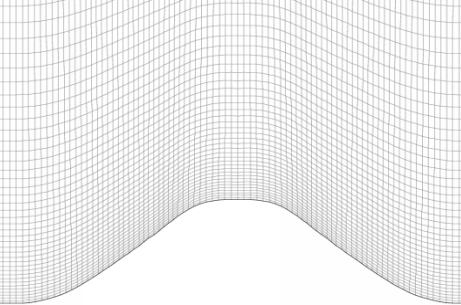Figure 2: Medium grid for code F.

On the other hand, the user of code E generated a nearly constant grid, with 3 meshes of 4,000; 16,000 and 64,000 nodes, so that the effect of mesh refinement is more visible (the near wall cells with code E and 64,000 nodes are nearly identical to code F with the 16,000 nodes grid).

 Code E Coarse Medium Fine Number of cells 4000 16000 64000 Dimensions 100×40 200×80 400×160 y+ (at inlet) 104 52 26

With code F, no difference between the 3 meshes is found until the top of the hill where it is seen that the vertical velocity component increases while the axial component maximum is decreased, both coming very close to the experiment.

The effect of mesh refinement is better illustrated with code E, on Figure 5, at the top of the hill, x = 0, and just at the reattachment, x = 05 (before the summit there is no effect). From the U and k profiles it is seen that most of the variation originates from the variation of the height of the first near wall cell. This explains why with code F and keeping this size constant hardly any change was observed. The variation of the V profiles are more important, and spread further away from the wall. This can be explained by the fact that as the peak in U velocity component at the summit decreases with mesh refinement, the vertical deflection of the streamlines must increase in order to conserve mass. Due to the elliptic nature of pressure, this effect spreads away further from the summit and is more visible on the V component scale, which is 10% of that of U.

The maximum of k at x = 05 is seen to be sensitive to mesh refinement. This was also where some differences were noted between the medium and fine mesh with code F. It is not surprising since the production of turbulence is very sensitive to the mean velocity gradients, and moreover the peak in the profile of k itself is naturally more sensitive to discretisation than the smoother mean velocity profile.

Effects of numerical schemes can be excluded by the fact that the 4 different codes yielded identical results on the finer grids. Calculations using minimal upwinding were less sensitive to grid refinement than those using pure upwind schemes, but differences were reduced with the finer grids. One simulation using the SMC with a purely centered convection scheme and with a second order resolution of the time-dependent equations yielded some vortex shedding and oscillation of the recirculation bubble, which may be physically significant.

### 5.2.2 Inlet Conditions

The effect of inlet conditions, imposed at 3 hill heights upstream of the hill summit, were tested with code F. Using either a constant profile for k based on turbulence intensity, k = 3/2 (Ui)2, or one fitted from the experimental data made no difference. Imposing a constant dissipation profile, corresponding to the average of ε across a fully developed channel flow, led to a dramatic overestimation of k on the hill summit. Using the length scale relation L=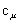3/4 k3/2 /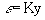led to a good agreement of velocity and k profiles with experiments at the hill summit.

With code E, analytical profiles based on a compilation of DNS and LES results of channel flows (AGARD) were defined as follows:

$k^{+}=0.07(y^{+}y^{+})\exp \left(-{\frac {y^{+}}{8}}\right)+4.5\left(1-\exp \left(-{\frac {y^{+}}{20}}\right)\right)\left({\frac {1}{{\frac {4y^{+}}{{\text{Re}}_{*}}}+1}}\right)$A value for the friction velocity of u* = 0.079 m/s is provided from the experimental data, also in accordance with usual correlations for friction coefficients or head losses in channel flow.

These profiles matched the experimental data at inlet quite well. In a later stage these same profiles were also adopted for code F, and yielded a small but visible improvement at the hill summit. These inlet conditions were subsequently used for all results and all codes shown herein. Such refined profiles would actually only be needed for testing low Re models, but were convenient here to ensure that the same inlet profiles were given for the 4 codes whatever the grids.

As concerns the SMC simulations, the same analytical k and ε profiles were used. Surprisingly no differences were found at the hill summit when using either isotropic or the algebraic stress model assumptions for anisotropy. It seems that the 3 hill heights after the inlet is sufficient to recover developed stress profiles while starting from the crude isotropic turbulence assumption.

### 5.2.3 Turbulence models

The constants of the k-ε model are given the usual values (Launder & Spalding (1974)):

 Cμ σk σε Cε1 Cε2 0.09 1.0 1.3 1.44 1.92

The SMC uses the standard Rotta return to isotropy pressure strain-term, and a linear isotropisation of production, with also standard values C1=1.8 and C2=0.6. For the turbulent transport terms, it should be noted that for the turbulent transport of the Re stresses, instead of the Daly and Harlow model used in codes E and G, code F uses a simple eddy viscosity with a Schmidt number of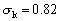(Lien and Leschziner ).